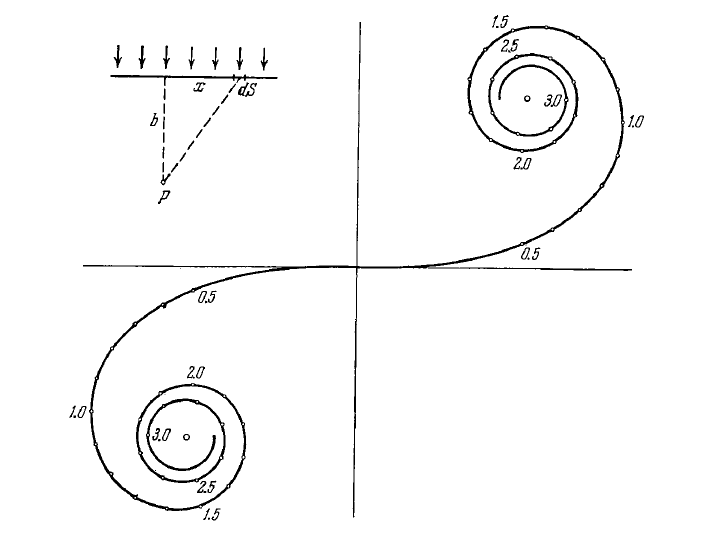# Diffraction of light

For page specific messages
For page author info
• Radius of the periphery of $kth$ Fresnel zone: $$r_k = \sqrt{k\lambda \frac{ab}{a+b} }, k=1, 2, 3, ...$$
• Cornu's spiral (Fig. 5.19). The numbers along that spiral correspond to the values of parameter $v$ . In the case of a plain wave $v = x\sqrt{2/b\lambda},$ where $x$ and $b$ are the distances defining the position of the element $dS$ of a wavefront relative to the observation point $P$ as shown in the upper left corner of the figure.• Fraunhofer diffraction produced by light falling normally from a slit. Condition of intensity minima: $$b\sin\theta = \pm k \lambda, k= 1,2,3,...,$$ where $b$ is the width of the slit, $\theta$ is the diffraction angle.
• Diffraction grating, with light falling normally. The main Fraunhofer maxima appear under the condition $$d \sin\theta = \pm k\lambda, k=0, 1, 2, ...,$$ the condition for additional maxima: $$d \sin\theta = \pm \frac{k^\prime}{N}\lambda,$$ where $k^\prime = 1, 2, ...$, except for $0, N, 2N,...$.
• Angular dispersion of a diffraction grating: $$D = \frac{\delta\theta}{\delta\lambda} = \frac{k}{d\cos\theta}.$$
• Resolving power of a diffraction grating: $$R = \frac{\lambda}{\delta\lambda}=kN,$$ where $N$ is the number of lines of the grating.
• Resolving power of an objective: $$R = \frac{1}{\delta\psi}=\frac{D}{1.22 \lambda},$$ where $\delta\psi$ is the least angular separation resolved by the objective, $D$ is the diameter of the objective.
• Bragg's equation. The condition of diffraction maxima: $$2d\sin\alpha = \pm k\lambda,$$ where $d$ is the interplanar distance, $\alpha$ is the glancing angle, $k=1,2,3,...$.

n
0

X